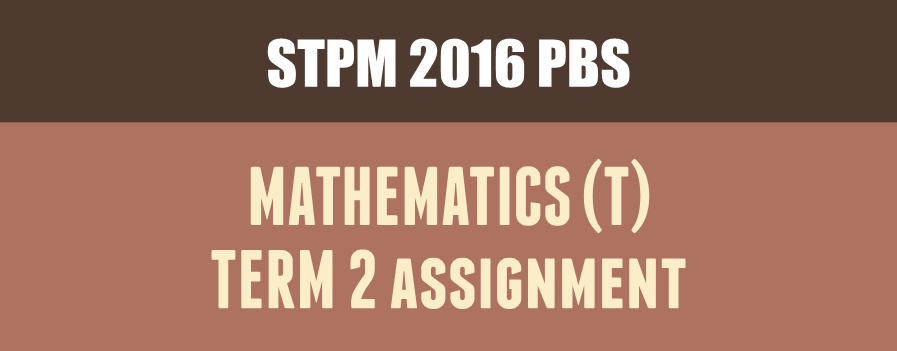#### kklee@kkleemaths.com#### Tuition Timetable

Check the latest timetable of my tuition classes.

#### Resources

Complete Resources about past year questions are here.

#### Ai Tuition

Visit Ai Tuition Website for more subjects tuition.

VIEW ALL SERVICES

Discussion –

4

Discussion –

4

# STPM 2016 Mathematics (T) Term 2 Assignment## Introduction

The concept of a limit plays a central role in calculus. For example, continuity, derivative and integral require this concept. In this assignment, you are required to explore the concept of a limit.

## Sample Question

Question 1

(a) Draw an equilateral triangle inscribed in a circle of radius$x$ cm. Express the area of the triangle$A_3$ in term of$x$.

(b) Repeat 1(a) with square, regular pentagon, regular hexagon, …, regular polygon with n sides in the circle of radius$x$ cm.

(c) Determine the value of$\frac{A_n}{x^2}$ correct to three decimal places when$n$ is large.

Question 2

A function$f_k$  is defined by$f_k(x) =(1+kx)^{\frac{1}{x}}$.

(a)(i) Tabulate the values$x$ and$f_1(x)$ when x equals to 0.1, 0.01, 0.001, …. Deduce value of$\lim_{x\to 0} f_1(x)$.

(a)(ii) Tabulate the values$x$ and$f_k(x)$ for$k = 2$, 3 and 4, when$x$ equals to 0.1, 0.01, 0.001, ….  Deduce value of$\lim_{x\to 0}f_k(x)$.

(b) Show that$\lim_{x\to\infty} \left(1+\frac{k}{x}\right)^x=\lim_{x\to 0}(1+kx)^\frac{1}{x}$.

Question 3

A function g is defined by$g(t) = \int_1^t \frac{1}{x(x+1)}dx$, where$t>1$.

(a) Suppose$t$ is an integer, estimate$g(t)$ using the trapezium rule with

(i)$t-1$ strips,

(ii)$2(t-1)$ strips,

(iii)$4(t-1)$ strips.

In each case, express$g(t)$ in terms of$t$ and determine $\lim_{t\to\infty} g(t)$.

(b) Suppose$t$ is a real number, find$g(t)$ using integration and determine $\lim_{t\to\infty} g(t)$.

##### Sample Solution
Sample solution will not be posted for this semester. Assignment sample solution will be continued only after this semester.

Dear my students, please join the my Facebook Group to get the full solution for Question 3 (a) (iii).

Here are our most recent updates posts

- Feel free to check it out -#### KK LEE

KK LEE has been a STPM Mathematics tuition teacher since June 2006, but his love of Maths dates back to at least 1999 when he was Form 4. KK LEE started teaching in 2006 at Pusat Tuisyen Kasturi. He was known as "LK" when he was teaching in PTK. After teaching STPM Mathematics for 8 years in PTK, he joined Ai Tuition Centre in 2014. Over the years he has taught Mathematics (T), Mathematics (S), Mathematics (M), Additional Mathematics.

1.Sir! Thank you for guiding us all these semesters!!! I’ve learned a lot from your solutions!
Sir, I do really appreciate your hard work! THANK YOU SIR!!

•Thanks

2.sir can you post the sample answer already? sem 2 already over , i need it as reference . Thank you in advance

•